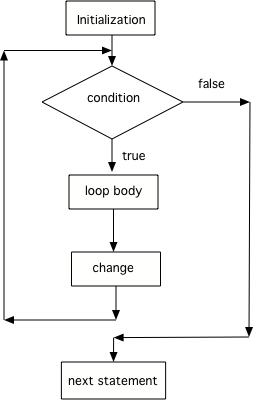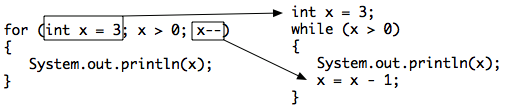# 4.2. For Loops¶

Another type of loop in Java is a for loop. This is usually used when you know how many times you want the loop to execute. It is often a simple counter-controlled loop to do the loop body a set number of times.

## 4.2.1. Three Parts of a For Loop¶

A for-loop combines all 3 parts of writing a loop in one line to initialize, test, and change the loop control variable. The 3 parts are separated by semicolons (`;`). Each of the three parts of a `for` loop declaration is optional (initialization, condition, and change), but the semicolons are not optional.

```for (initialize; test condition; change)
{
loop body
}
```

The for-loop is almost a shortcut way to write a while loop with all three steps that you need in one line. One of the strange things about a `for` loop is that the code doesn’t actually execute where you see it in the declaration. The code in the initialization area is executed only one time before the loop begins, the test condition is checked each time through the loop and the loop continues as long as the condition is true, and the loop control variable change is done at the end of each execution of the body of the loop, just like a while loop. When the loop condition is false, execution will continue at the next statement after the body of the loop.Figure 2: Control flow in a for loop¶

You can compare a `while` loop to a `for` loop to understand that a `for` loop actually executes like a `while` loop does if you use the `while` loop to repeat the body of the loop a specific number of times.Figure 3: Showing how a for loop maps to a while loop¶

Here is a for loop that counts from 1 to 5. Can you change it to count from 2 to 10?

Here is a while loop that counts from 5 to 10. Run it and see what it does. Can you change it to a for-loop? Run your for-loop. Does it do the same thing?

Note

Two common patterns in for-loops are to count from 0 up to an number (using <) or count from 1 to the number including the number (using <=). Remember that if you start at 0 use <, and if you start at 1, use <=. The two loops below using these two patterns both run 10 times. The variable i (for index) is often used as a counter in for-loops.

```// These loops both run 10 times
// If you start at 0, use <
for(int i = 0; i < 10; i++)
{
System.out.println(i);
}
// If you start at 1, use <=
for(int i = 1; i <= 10; i++)
{
System.out.println(i);
}
```Check your understanding

The following method has the correct code to print out all the even values from 0 to the value of 10, but the code is mixed up. Drag the blocks from the left into the correct order on the right and indent them correctly. Even though Java doesn’t require indention it is a good habit to get into. You will be told if any of the blocks are in the wrong order or not indented correctly when you click the “Check Me” button.

## 4.2.2. Decrementing Loops¶

You can also count backwards in a loop starting from the last number and decrementing down to 0 or 1. All 3 parts of the loop must change to count backwards including the test of when to stop. For example, “for (int i=5; i > 0; i–)`` counts from 5 down to 1.

What do you think will happen when you run the code below? How would it change if you changed line 11 to initialize i’s value to 3? Try the Code Lens button to visualize and trace through this code.

The method printPopSong prints the words to a song. It initializes the value of the variable i equal to 5 and then checks if i is greater than 0. Since 5 is greater than 0, the body of the loop executes. Before the condition is checked again, i is decreased by 1. When the value in i is equal to 0 the loop stops executing.

Can you make the loop count by 2s backwards? It should print out 5 3 1? Remember to change all 3 parts of the for loop. Use the CodeLens if you need to debug your code.

## 4.2.3. Turtle Loops¶

Do you remember when we used the turtle objects to draw shapes? To create a square without loops we had to repeat code to go forward and turn 90 degrees to the right 4 times like below. Can you change the code below to remove the repeated lines of code and use a loop to draw 4 sides of the square? Did you notice that the code becomes a lot shorter? You should only need 1 forward and 1 turn command in the loop. Whenever you find yourself repeating code, try to use a loop instead!

(If the code below does not work for you, you can copy the code into this repl.it link (refresh page after forking and if it gets stuck) or download the files here to use in your own IDE.)

Can you change the code below to remove the repeated lines of code and use a loop to draw 4 sides of the square?

## 4.2.4.Programming Challenge : Turtles Drawing Shapes¶

In the last exercise, you used a for-loop to have the turtle draw a square. Use the Active Code window below or this repl.it link to have yertle draw the following shapes using loops.

1. Have yertle draw an equilateral triangle using a loop. How many times should the loop run? Remember that it ran 4 times for a square, so how many for a triangle? What angle should you use for the turns? One way to figure this out is to notice that to complete a shape, all the exterior angles should add up to 360 degrees. So, for a square 4x90 = 360.

2. Have yertle draw a pentagon using a loop. A pentagon has 5 sides. What external angle should you use for the turns? Remember they have to add up to 360 degrees.

3. Create a variable n that holds the number of sides for any polygon, and use n in your loop. Can you have the loop draw a variety of shapes by just changing the value of the variable n? The power of abstraction! Can you draw a 9 sided nonagon? (Note that if the turtle runs into walls, it stays there and will mess up the shape, so you may have to move the turtle or go forward smaller amounts).

Use a for-loop to draw a triangle. Then, change it to a pentagon. Then change it to draw any polygon using a variable n that holds the number of sides. Note that the angles in the turns have to add up to 360.

## 4.2.5. Summary¶

• There are three parts in a for loop header: the initialization, the test condition (a Boolean expression), and an increment or decrement statement to change the loop control variable.

• In a for loop, the initialization statement is only executed once before the evaluation of the test Boolean expression. The variable being initialized is referred to as a loop control variable.

• In each iteration of a for loop, the increment or decrement statement is executed after the entire loop body is executed and before the Boolean expression is evaluated again.

• A for loop can be rewritten into an equivalent while loop and vice versa.

You have attempted of activities on this page